previous lesson

Dispersion IQV

INDEX OF QUALITATIVE VARIATION: (for Nominal and discrete Ordinal Data)

IQV measures the ratio of the actual variation divided by the highest possible variation

IQV=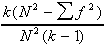where: k = # of categories

N = # of cases

f2 = sum of the squared frequencies

Example:

 ABORTIONS f f2 Always wrong 6 36 Wrong except self defense 1 1 Wrong except S.D. & rape 9 81 Personal choice of both parents 31 961 Woman's choice 25 625 N = 75 1,704

Q: What is spread or variation in abortion attitudes?

A: IQV =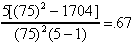SOURCE: STUDENT SURVEY SDSU 1995

Interpretation: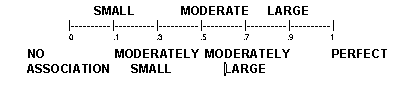B. Interval Data and continuous Ordinal Data: Range

Range is found by subtracting the lower true limit of the lowest category from the upper true limit of the highest category.

Example: Ages of Sociology 201 Students

 Ages (NB) lower true limit upper true limit f cum f 18-22 17.50 22.499 54 54 23-27 22.50 27.499 13 67 28-32 27.50 32.499 3 70 33-37 32.50 37.499 3 73 38-42 37.50 42.499 1 74 74 = N

SOURCE: STUDENT SURVEY SDSU 1995

Example: 42.499 - 17.50 = 25 The range of this sample is 25 years.

C. Inter Quartile Range: measure the range of the middle 50% .

IQR = P75 - P25 where: (note the 75 to 25 could be any range but this specifies the middle 50%)

P75 =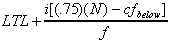P25 =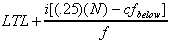cfbelow = cumulative frequency below interval containing the percentile.

f = the number of cases in the interval containing the percentile.

i = width of interval

N = total number in sample

LTL = lower true limit of the interval containing the percentile.

P75 =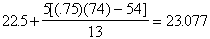P25 =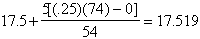23.077 - 17.519 = 5.56 years